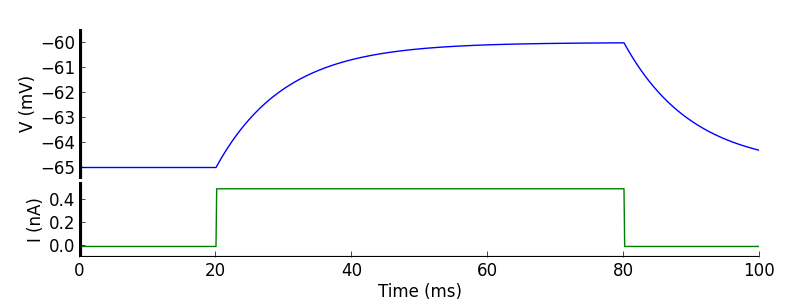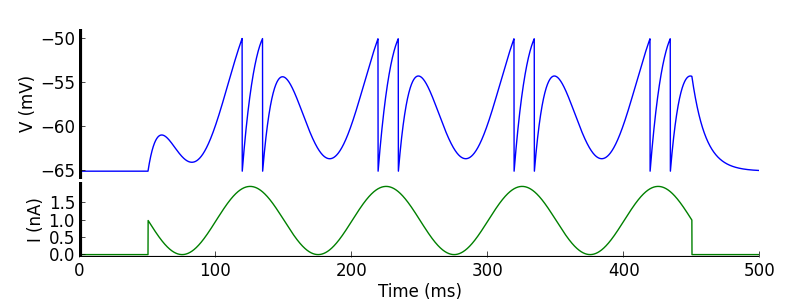# Injecting current¶

Current waveforms are represented in PyNN by `CurrentSource` classes. There are four built-in source types, and it is straightforward to implement your own.

There are two ways to inject a current waveform into the cells of a `Population`, `PopulationView` or `Assembly`: either the `inject_into()` method of the `CurrentSource` or the `inject()` method of the `Population`, `Assembly`, etc.

```>>> pulse = DCSource(amplitude=0.5, start=20.0, stop=80.0)
>>> pulse.inject_into(population[3:7])
``````>>> sine = ACSource(start=50.0, stop=450.0, amplitude=1.0, offset=1.0,
...                 frequency=10.0, phase=180.0)
>>> population.inject(sine)
``````>>> steps = StepCurrentSource(times=[50.0, 110.0, 150.0, 210.0],
...                           amplitudes=[0.4, 0.6, -0.2, 0.2])
>>> steps.inject_into(population[(6,11,27)])
``````>>> noise = NoisyCurrentSource(mean=1.5, stdev=1.0, start=50.0, stop=450.0, dt=1.0)
>>> population.inject(noise)
```For a full description of all the built-in current source classes, see the API reference.

Todo

write “implementing-your-own-current-source” (e.g., implement “chirp”)